SSC BOARD PAPERS IMPORTANT TOPICS COVERED FOR BOARD EXAM 2024

### Practice Set 3.5 Polynomials Class 9th Mathematics Part I MHB Solution

Practice Set 3.5 Polynomials Class 9th Mathematics Part I MHB Solution

###### Practice Set 3.5
Question 1.

Find the value of polynomial using given values for x.

i. x = 3 ii. x = -1

iii. x=0

The given polynomial is as follows:

2x – 2x3 + 7

(i) Put x = 3 in the given polynomial

= 2 × 3 – 2 × (3)3 + 7

= 6 – 2 × 27 + 7

= 6 -54 + 7

= -41

(ii) Put x = -1 in the given polynomial

= 2 × (-1) – 2 × (-1)3 + 7

= -2 + 2 + 7

= 7

(iii) Put x = 0 in the given polynomial

= 2 × 0 – 2 × (0)3 + 7

= 0 + 0 + 7

= 7

Question 2.

For each of the following polynomial, find and

p(x) = x3

To find: p (1)

Put x = 1 in the given polynomial

p (1) = (1)3

p (1) = 1

To find: p (0)

Put x = 0 in the given polynomial

p (0) = 0

To find: p (-2)

Put x = -2 in the given polynomial

p (-2) = (-2)3

p (-2) = -8

Question 3.

For each of the following polynomial, find and

p(y) = y2 – 2y + 5

To find: p (1)

Put x = 1 in the given polynomial

p (1) = (1)2 – 2 × 1 + 5

= 1 – 2 + 5

= -1 + 5

= 4

To find: p (0)

Put x = 0 in the given polynomial

p (0) = 0 – 0 + 5

= 5

To find: p (-2)

Put x = -2 in the given polynomial

p (-2) = (-2)2 – 2 × (-2) + 5

= 4 + 4 + 5

p (-2) = 13

Question 4.

For each of the following polynomial, find and

p(x) = x4 – 2x2 - x

To find: p (1)

Put x = 1 in the given polynomial

p (1) = (1)4 – 2 × (1)2 – 1

= 1 – 2 – 1

p (1) = -2

To find: p (0)

Put x = 0 in the given polynomial

p (0) = 0 – 0 – 1

p (0) = -1

To find: p (-2)

Put x = -2 in the given polynomial

p (-2) = (-2)4 – 2 × (-2)2 – 1

= 16 – 2 × 4 – 1

= 16 -8 -1

= 7

Question 5.

If the value of the polynomial m3 + 2m + a is 12 for m =2, then find the value of a.

Put m = 2 throughout the polynomial

(2)3 + 2 × 2 + a = 12

8 + 4 + a = 12

12 + a = 12

a = 0

Question 6.

For the polynomial mx2 + 2x + 3 if p(-1) = 7 then find m.

Put x = -1 throughout the polynomial

m × (-1)2 – 2 × (-1) + 3 = 7

m + 2 + 3 = 7

m + 5 = 7

m = 7-5

m = 2

Question 7.

Divide the first polynomial by the second polynomial and find the remainder using factor theorem.

(x2 – 7x + 9); (x + 1)

The coefficient form of the first polynomial is as follows:

⟹ 1.x2 – 7.x + 9

⟹ (1, -7, 9)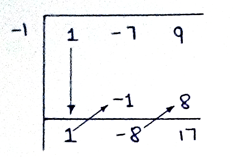Therefore, the remainder is = 17

Question 8.

Divide the first polynomial by the second polynomial and find the remainder using factor theorem.

(2x3 – 2x2 + ax – a); (x-a)

The coefficient form of the first polynomial is as follows:

⟹ 2.x3 – 2.x2 + a.x – a

(2, -2, a, -a)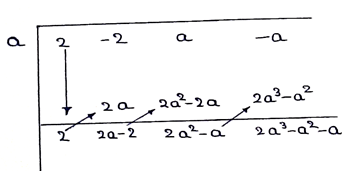The remainder is = 2a3-a2 –a

Question 9.

Divide the first polynomial by the second polynomial and find the remainder using factor theorem.

(54m3 + 18m2 – 27m + 5); (m-3)

The coefficient form of the first polynomial is as follows:

⟹ 54.m3 + 18.m2 – 27.m + 5

(54, 18, -27, 5)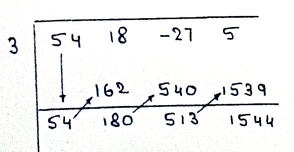The last term in the synthetic division is the remainder of the given division method.

The remainder is =1544.

Question 10.

If the polynomial y3 – 5y2 + 7y + m is divided by y+2 and the remainder is 50 then find the value of m.

Put y = -2 throughout the given polynomial

(-2)3 – 5 × (-2)2 + 7 × (-2) + m = 50

-8 – 20 -14 + m = 50

-28 + 14 + m = 50

-14 + m = 50

m = 50 + 14

m = 64

Question 11.

Use factor theorem to determine whether x+3 is factor of x2 + 2x – 3 or not.

The coefficient form of the polynomial:

⟹ 1.x2 + 2.x – 3

(1, 2, -3)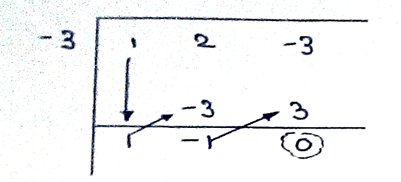Since the last term is zero which indicates that the polynomial on dividing by x + 3 leaves no remainder and hence x + 3 is the factor of x2 + 2x – 3

Question 12.

If (x-2) is a factor of x3 – mx2 + 10x - 20 then find the value of m.

Put x = 2 throughout the polynomial and equate it to zero as x -2 is the factor of the given polynomial.

(2)3 – m × (2)2 + 10 × 2 – 20 = 0

8 – 4m + 20 – 20 = 0

4m = 8

m = 2

So for x-2 to be the factor of the given polynomial, the value of m = 2.

Question 13.

By using factor theorem in the following examples, determine whether q(x) is a factor p(x) or not.

p(x) = x3 – x2 – x – 1, q(x) = x - 1

The coefficient form of p(x) is:

p(x) = 1.x3 – 1.x2 – 1.x – 1

(2, -1, 0, -45)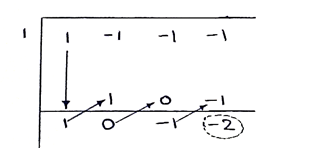Since the last term is not term is not zero, q(x) is not the factor of p(x).

Question 14.

By using factor theorem in the following examples, determine whether q(x) is a factor p(x) or not.

p(x) = 2x3 – x2 – 45, q(x) = x - 3

The coefficient form of p(x) is:

p(x) = 2.x3 – 1.x2 + 0.x – 45

(2, -1, 0, -45)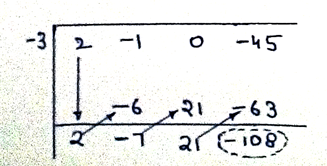Since the last term is not term is not zero, q(x) is not the factor of p(x).

Question 15.

If (x31 + 31) is divided by (x+1) then find the remainder.

Put x= -1 throughout the polynomial

x(-1) = (-1)31 + 31

= -1 + 31

= 30

So the remainder is 30.

Question 16.

Show that m-1 is a factor of m21 - 1 and m22 - 1

Put m = 1 in the first polynomial

m (1) = (1)21 – 1

= 1 – 1

= 0

So m-1 is the factor of m21 -1

Put m = 1 in the second polynomial

m (1) = (1)22 – 1

= 1 – 1

= 0

So m-1 is the factor of m22 -1

Question 17.

If x-2 and x – 1/2 both are the factors of the polynomial nx2 – 5x + m, then show that m= n=2

Put x = 2 in the given polynomial:

n× 4 – 5× 2 + m = 0

4n + m = 10 ……………………………. (i)

Put x = 1/2 in the given polynomial

n × 1/4 - 5 × 1/2 + m = 0

n + 4m – 10 = 0

n + 4m = 10 ……………………………….. (ii)

Since the RHS of both the equations is same,

4n + m = n + 4m

3n = 3m

n = m

Put n = m in the equation (i)

4m + m = 10

5m = 10

m = 2 = n

Question 18.

If p(x) = 2+5x then p(2) + p(-2)-p(1)

Put x = 2 in the polynomial

p(2) = 2 + 5× 2

= 2 + 10

= 12

Put x = -2 in the polynomial

p(-2) = 2 + (5× -2)

= 2 – 10

= -8

Put x = 1 in the given polynomial

P(1) = 2 + 5× 1

= 2 + 5

= 7

p(2) + p(-2) – p(1) = 12 – 8 - 7

= -3

Question 19.

If p(x)=2x2-5√3+5 then p(5√3).

Put x = 5√3 in the given polynomial

p(5√3) = 2×(5√3)2 – 5√3 × 5√3 + 5

= 2 × 25 × 3 – 25 × 3 + 5

= 150 – 75 + 5

p (5√3) = 80